Question

# Two spherical objects are separated by a distance of 1.07 x 102m. The objects are initially...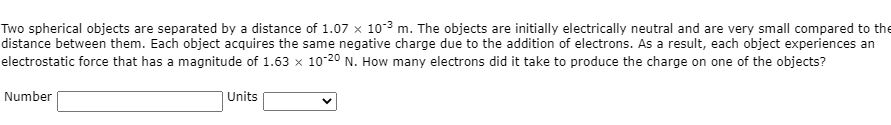Two spherical objects are separated by a distance of 1.07 x 102m. The objects are initially electrically neutral and are very small compared to the distance between them. Each object acquires the same negative charge due to the addition of electrons. As a result, each object experiences an electrostatic force that has a magnitude of 1.63 x 10-20 N. How many electrons did it take to produce the charge on one of the objects? Number Units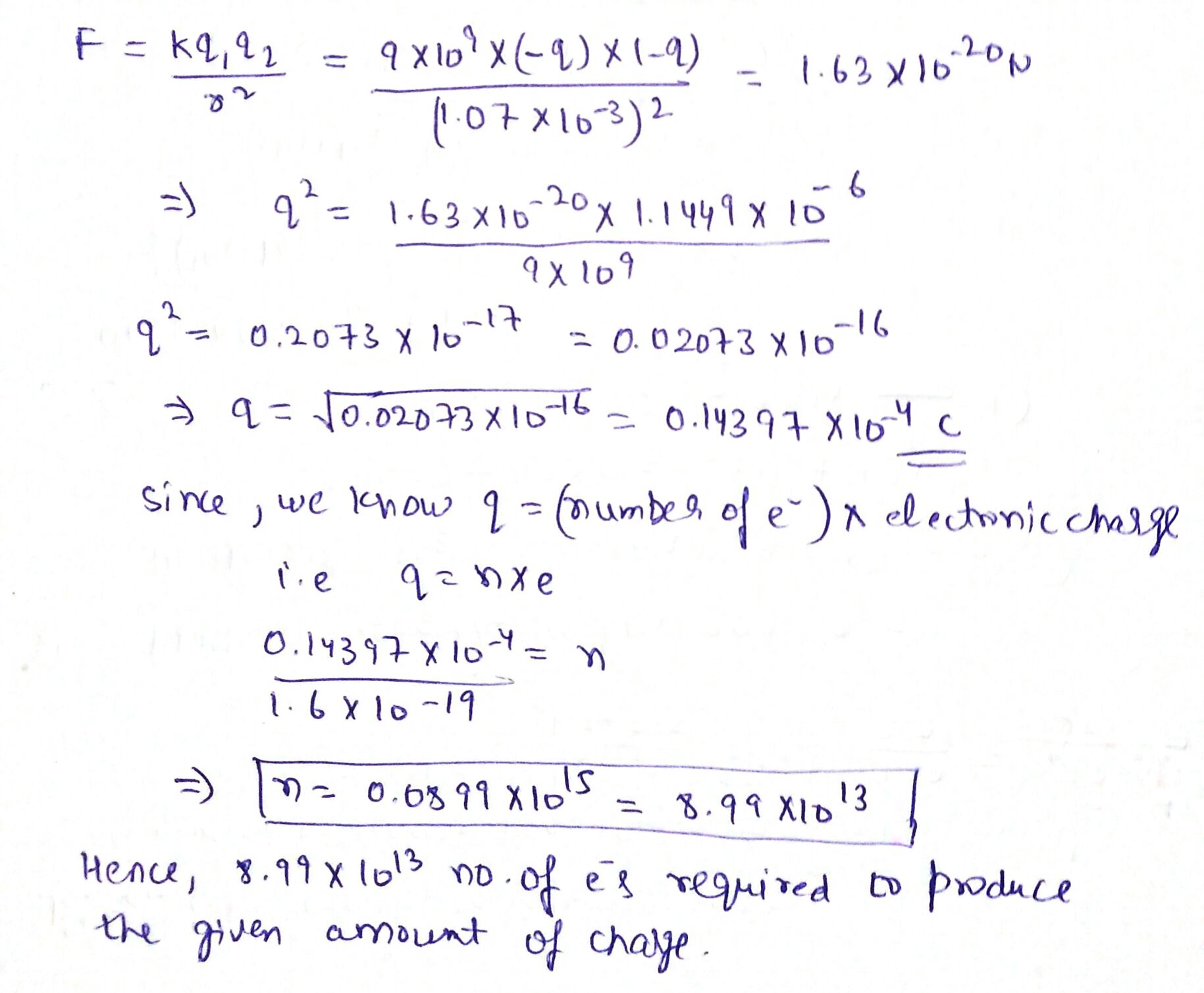#### Earn Coins

Coins can be redeemed for fabulous gifts.

Similar Homework Help Questions
• ### Two spherical objects are separated by a distance of 1.25 × 10-3 m. The objects are...

Two spherical objects are separated by a distance of 1.25 × 10-3 m. The objects are initially electrically neutral and are very small compared to the distance between them. Each object acquires the same negative charge due to the addition of electrons. As a result, each object experiences an electrostatic force that has a magnitude of 1.78 × 10-20 N. How many electrons did it take to produce the charge on one of the objects?

• ### Two spherical objects are separated by a distance of 1.84 × 10-3 m. The objects are...

Two spherical objects are separated by a distance of 1.84 × 10-3 m. The objects are initially electrically neutral and are very small compared to the distance between them. Each object acquires the same negative charge due to the addition of electrons. As a result, each object experiences an electrostatic force that has a magnitude of 8.23 × 10-21 N. How many electrons did it take to produce the charge on one of the objects?

• ### Two spherical objects are separated by a distance of 2.04 × 10-3 m. The objects are...

Two spherical objects are separated by a distance of 2.04 × 10-3 m. The objects are initially electrically neutral and are very small compared to the distance between them. Each object acquires the same negative charge due to the addition of electrons. As a result, each object experiences an electrostatic force that has a magnitude of 1.08 × 10-20 N. How many electrons did it take to produce the charge on one of the objects?​

• ### Two spherical objects are separated by a distance of 1.47 × 10-3 m. The objects are...

Two spherical objects are separated by a distance of 1.47 × 10-3 m. The objects are initially electrically neutral and are very small compared to the distance between them. Each object acquires the same negative charge due to the addition of electrons. As a result, each object experiences an electrostatic force that has a magnitude of 1.53 × 10-20 N. How many electrons did it take to produce the charge on one of the objects?

• ### Two spherical objects are separated by a distance that is 9.00 10-3 m. The objects are...

Two spherical objects are separated by a distance that is 9.00 10-3 m. The objects are initially electrically neutral and are very small compared to the distance between them. Each object acquires the same negative charge due to the addition of electrons. As a result, each object experiences an electrostatic force that has a magnitude of 1.1375 10-21 N. How many electrons did it take to produce the charge on one of the objects? electrons?

• ### Two spherical objects are separated by a distance of 1.01 × 10-3 m. The objects are initially electrically neutral and are very small compared to the distance between them. Each object acquires the sa...

Two spherical objects are separated by a distance of 1.01 × 10-3 m. The objects are initially electrically neutral and are very small compared to the distance between them. Each object acquires the same negative charge due to the addition of electrons. As a result, each object experiences an electrostatic force that has a magnitude of 1.83 × 10-20 N. How many electrons did it take to produce the charge on one of the objects?

• ### Twe spherical objects are separated by a distance of 2.01 x 10 m. The objects are...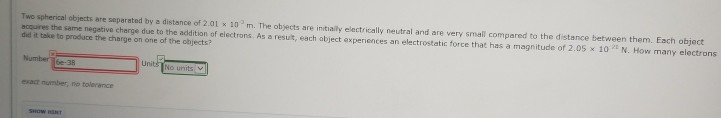Twe spherical objects are separated by a distance of 2.01 x 10 m. The objects are initialy elactrically neutral and are very small compared to the distance between them. Each object acquires the same negetive charge due to the addition of electrons. As a resut, each abject experiences an electrostatic force that has a magnitude of 2.05 x 10 N. How many electrons did it take to produce the charge on one of the objects? exat number, no tolerance

• ### CJ10 18 P.009 Two spherical objects are separated by a distance that is 5.40 x 103...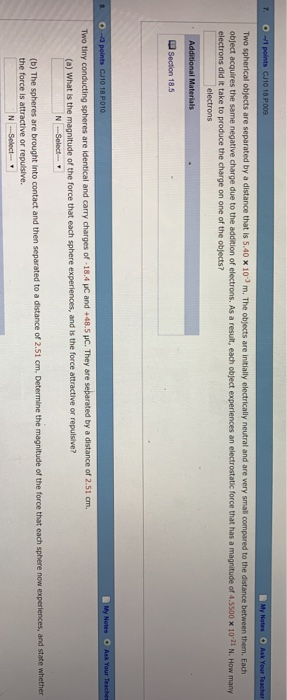CJ10 18 P.009 Two spherical objects are separated by a distance that is 5.40 x 103 m. The objects are initially electrically neutral and are very small o ect acquires the same negative charge due to the addition of electrons. As a result, each object experiences an electrostatic force that has a magnitude of 4 SS00 x 10-21 N How many electrons did it take to produce the charge on one of the objects? electrons CJ10 18 P010 Two tinyc...

• ### Do each question separately and show step by step Two spherical objects are separated by a...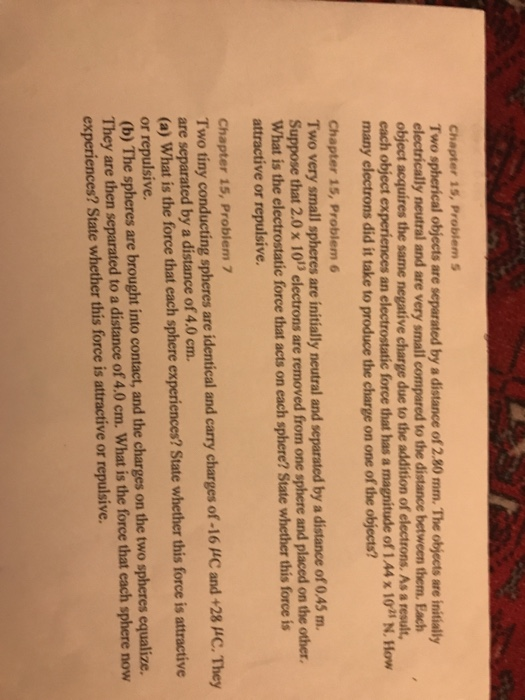Do each question separately and show step by step Two spherical objects are separated by a distance of 2.80 mm. The objects are initially electrically neutral and are very small compared to the distance between them. Each object acquires the same negative charge due to the addition of electrons, As a result, each object experiences an electrostatic force that has a magnitude of 1.44 times 10^-25 N. How many electrons did it take to produce the charge on one of...

• ### physics

Two spherical objects are separated by a distance of 2.84 x 10-3 m. The objects are initially electrically neutral and are very small compared to the distance betweenthem. Each object acquires the same negative charge due to the addition of electrons. As a result, each object experiences an electrostatic force that has a magnitudeof 2.31 × 10-21 N. How many electrons did it take to produce the charge on one of the objects?